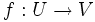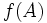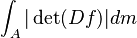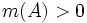Diffeomorphism implies nullset-preserving

Statement

Let$U$ and$V$ be open subsets in$\R^n$, and let$f:U \to V$ be a diffeomorphism (i.e.$f$ is a smooth map and the inverse of$f$ is also smooth). Then,$f$ is a nullset-preserving map, in the sense that a subset$A$ of$U$ has Lebesgue measure zero iff$f(A)$ has Lebesgue measure zero.

Proof

Proof idea

The idea behind the proof is the change-of-variables formula: namely, that for any$A$, the measure of$f(A)$ is given by:$\int_A |\det(Df)| dm$

Because the map is invertible, the determinant is everywhere nonzero, so we are integrating a strictly positive function on$A$. The integral of this function is positive iff$m(A) > 0$.

Note that the proof only requires the map to be$C^1$ and have a$C^1$ inverse; in fact, even more weakly, we only require the map to be Lipschitz and have a Lipschitz inverse.